# Trigonometric Functions Chapter Exam

Exam Instructions:

Choose your answers to the questions and click 'Next' to see the next set of questions. You can skip questions if you would like and come back to them later with the yellow "Go To First Skipped Question" button. When you have completed the practice exam, a green submit button will appear. Click it to see your results. Good luck!

Answered 0 of 5

### Page 1

#### Question 1 1. Simplify the following expression.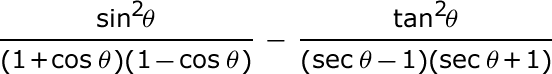#### Question 2 2. Simplify the following expression.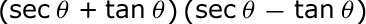#### Question 3 3. What's the value of sec2 (θ) given that the equation below is true?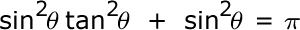#### Question 4 4. What is the value of cos(θ) given that the equation below is true?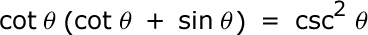#### Question 5 5. What's the value of 2sin(θ)cos(θ) given that the equation below is true?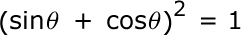#### Trigonometric Functions Chapter Exam Instructions

Choose your answers to the questions and click 'Next' to see the next set of questions. You can skip questions if you would like and come back to them later with the yellow "Go To First Skipped Question" button. When you have completed the practice exam, a green submit button will appear. Click it to see your results. Good luck!

Support